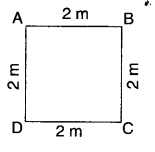• #### 1.  Perimeter of a rectangle =

• 2 × (Length + Breadth)
• 2 × (Length × Breadth)
• #### 2.  Perimeter of a square =

• 4 × Length of a side
• 2 × Length of a side
• 3 × Length of a side
• 6 × Length of a side
• #### 3.  Perimeter of an equilateral triangle

• 2 × Length of a side
• 3 × Length of a side
• 4 × Length of a side
• 6 x Length of a side
• #### 4.  Area of a rectangle =

• 2 × (Length + Breadth)
• 2 × (Length × Breadth)
• #### 5.  Area of a square =

• side × side
• 4 × Length of a side
• 2 × Length of a side
• 6 × Length of a side
• #### 6.  Perimeter of a regular pentagon =

• 4 × Length of a side
• 3 × Length of a side
• 6 × Length of a side
• 5 × Length of a side
• #### 7.  Perimeter of a regular hexagon =

• 3 × Length of a side
• 4 × Length of a side
• 5 × Length of a side
• 6 × Length of a side

• 30 m
• 60 m
• 20 m
• 10 m

• 12m
• 14m
• 24 m
• 7 m
• #### 10.  The perimeter of the figure is• 8m
• 16m
• 4m
• none of these
Report Question
Practice More On Mensuration
access_time